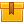# Percent, Ratio, and Proportion (A4304)

## Prerequisites

This lesson is designed to build on the skills presented in the third lesson of this program, Fractions.

## Description

In this lesson, participants learn how to draw comparisons between one amount and another. The concepts of percent, percentage, and base are taught and the relationship of these concepts to each other. Participants also learn how to find percent, percentage, and base by using paper and pencil as well as with a calculator. In addition, the lesson explains the concepts of ratio and proportion. This lesson teaches how to identify and construct both a ratio and proportion. Participants also learn how to use proportions to convert measurements from English to metric units.

## Objectives

Understand how words are translated into numerical values. Describe the concepts of base, percent, and percentage. Compare fractions and decimals to percentages. Understand the concept of percentage and recognize examples. Calculate percentage with paper and pencil. Use the calculator to find percentage. Understand the concept of percent and recognize examples. Calculate percent with paper and pencil. Use the calculator to find percent. Understand the concept of base and recognize examples. Calculate base with paper and pencil. Use the calculator to find base. Describe the concept of a ratio. Relate ratios to fractions. Given comparative data, construct ratios. Describe the concept of proportion. Find an unknown value in a proportion. Use proportions to convert measurements from one unit to another.

## ContentIn this lesson, participants learn how to draw comparisons between one
amount and another. The concepts of percent, percentage, and base are
taught and the relationship of these concepts to each other.
Participants also learn how to find percent, percentage, and base by
using paper and pencil as well as with a calculator. In addition, the
lesson explains the concepts of ratio and proportion. This lesson
teaches how to identify and construct both a ratio and proportion.
Participants also learn how to use proportions to convert measurements
from English to metric units.

•Percent, Ratio and Proportion

0
0 Reviews